Author: Oscar Cronquist Article last updated on September 29, 2022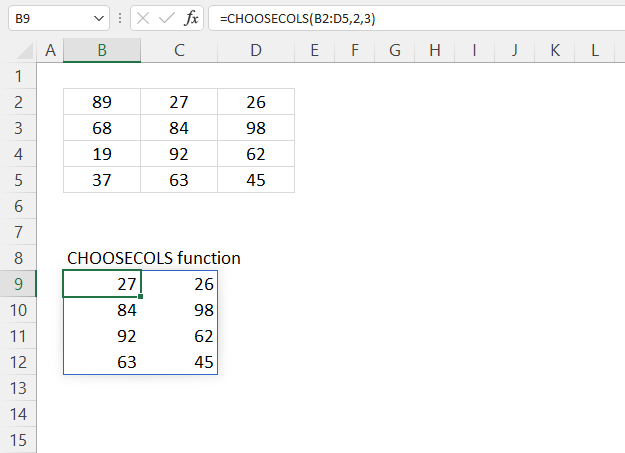The CHOOSECOLS function returns given columns from a cell range or array.

Dynamic array formula in cell B9:

=CHOOSECOLS(B2:D5, 2, 3)

The CHOOSECOLS function is available to Excel 365 users and is in the "Array manipulation" category.

## 1. CHOOSECOLS Function Syntax

CHOOSECOLS(array, col_num1, [col_num2], …)

## 2. CHOOSECOLS Function Arguments

 array Required. The source cell range or array. col_num1 Required. A number represents the column in a given cell range. [col_num2] Optional. Extra column numbers to be extracted.

## 3. CHOOSECOLS Function example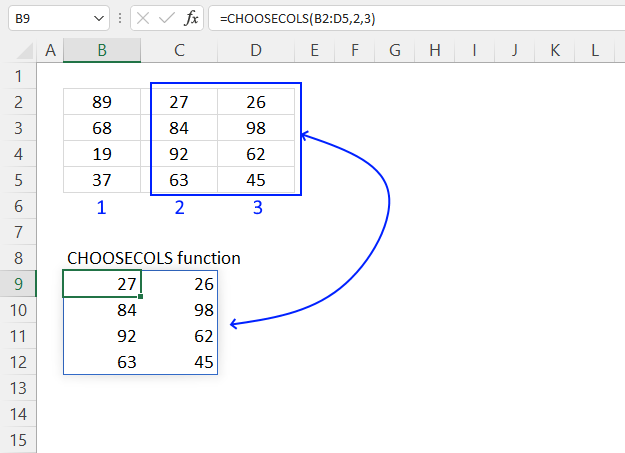The picture above shows how the CHOOSECOLS function extracts the second and third column from cell range B2:D5.

Dynamic array formula in cell B9:

=CHOOSECOLS(B2:D5, 2, 3)
Note! If any of the values in the source range change, the values in the CHOOSECOLS function output change as well.

### 3.1 Explaining formula

#### Step 1 - CHOOSECOLS function

CHOOSECOLS(array, col_num1, [col_num2], …)

array - B2:D5
col_num1 - 2
[col_num2] - 3

#### Step 3 - Evaluate function

CHOOSECOLS(B2:D5, 2, 3)

becomes

CHOOSECOLS({89, 27, 26;
68, 84, 98;
19, 92, 62;
37, 63, 45}, 2, 3)

and returns

{27, 26; 84, 98;
92, 62; 63, 45}

## 4. How to combine specific cell range columns - CHOOSECOLS Function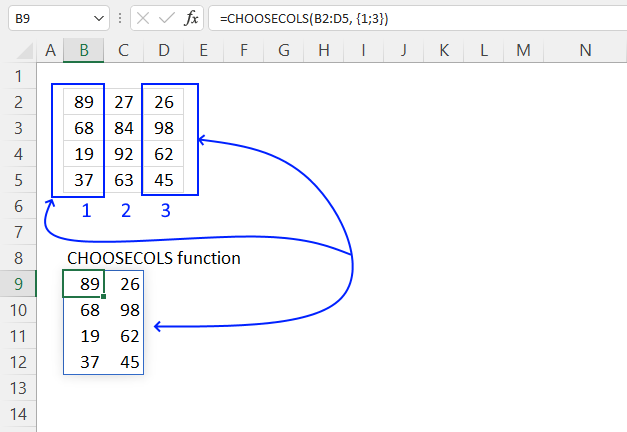The CHOOSECOLS function lets you use an array in the arguments, the image above shows an array in the second argument.

Dynamic array formula in cell B9:

=CHOOSECOLS(B2:D5, {1; 3})

### Explaining formula

#### Step 1 - Populate the array

The curly brackets let you build an array that you can use in most but not all Excel functions. The ; semicolon is a delimiting character that separates values row by row.

The CHOOSE function requires numbers separated with semicolons or whatever row delimiting character you use.

{1; 3}

#### Step 2 - CHOOSECOLS function

CHOOSECOLS(array, col_num1, [col_num2], …)

CHOOSECOLS(B2:D5, {1; 3})

becomes

CHOOSECOLS({89, 27, 26;
68, 84, 98;
19, 92, 62;
37, 63, 45}, {1; 3})

and returns

{89, 26;
68, 98;
19, 62;
37,45}

## 5. How to combine specific columns based on a given value - CHOOSECOLS Function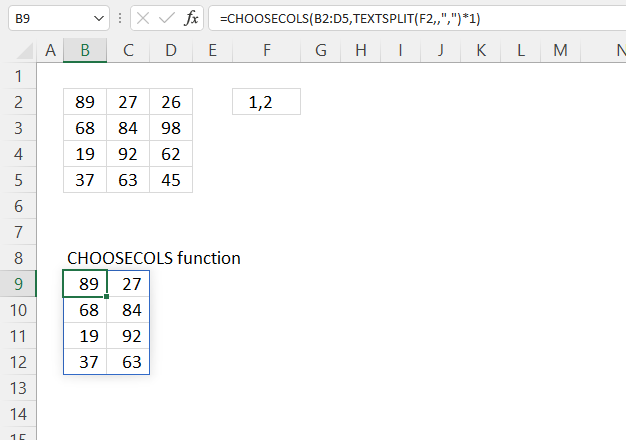The section shows how to return specific rows based on a string containing numbers. The TEXTSPLIT function separates values into an array.

Dynamic array formula in cell B9:

=CHOOSECOLS(B2:D5, TEXTSPLIT(F2, , ",")*1)

### Explaining formula

#### Step 1 - Split text

The TEXTSPLIT function lets you split a string into an array across columns and rows based on delimiting characters.

TEXTSPLIT(Input_Textcol_delimiter, [row_delimiter], [Ignore_Empty])

TEXTSPLIT(F2,,",")

becomes

TEXTSPLIT("1,2", , ",")

and returns {"1"; "2"}.

Note the double quotes, Excel handles these values as text values. The next step converts text values to numbers.

#### Step 2 - Convert text to numbers

The asterisk lets you multiply numbers in an Excel formula, I am using it here to convert text to numbers.

TEXTSPLIT(F2,,",")*1

becomes

{"1"; "2"}*1

and returns {1; 2}.

#### Step 3 - CHOOSECOLS function

CHOOSECOLS(B2:D5,TEXTSPLIT(F2,,",")*1)

becomes

CHOOSECOLS(B2:D5, {1; 2})

becomes

CHOOSECOLS({89, 27, 26;
68, 84, 98;
19, 92, 62;
37, 63, 45}, {1; 2})

and returns

{89, 27;
68, 84;
19, 92;
37, 63}

## 6. How to combine columns using negative values - CHOOSECOLS Function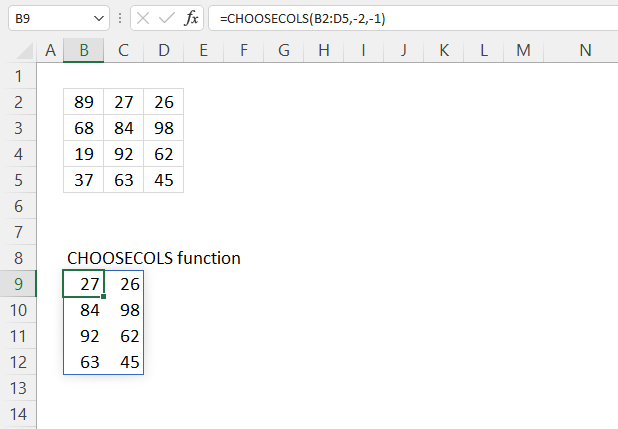The CHOOSECOLS function lets you use negative numbers, this will make the function count from the right.

Formula in cell B9:

=CHOOSECOLS(B2:D5, -2, -1)

CHOOSECOLS(array, col_num1, [col_num2], …)

## 7. CHOOSECOLS function returns a #VALUE! error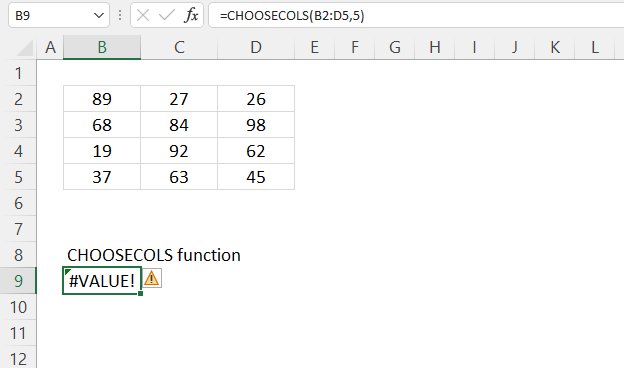The CHOOSECOLS function returns a #VALUE! error when no values can be displayed. There are only three columns in cell range B2:D5.

## 8. Extract values from given columns and return the list in random order - CHOOSECOLS function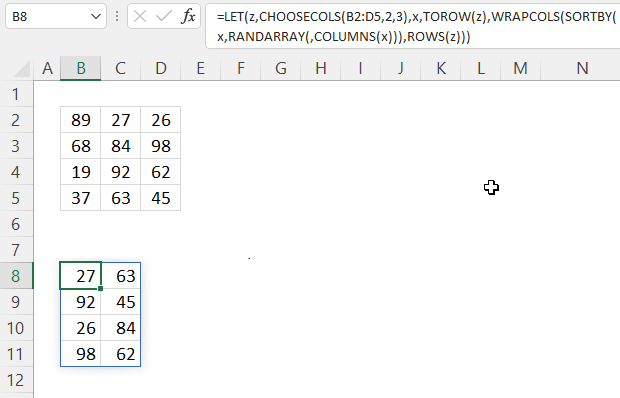The image above shows a formula that returns values in random order from columns two and three.

Dynamic array formula in cell B8:

=LET(z, CHOOSECOLS(B2:D5, 2, 3), x, TOROW(z), WRAPCOLS(SORTBY(x, RANDARRAY(, COLUMNS(x))), ROWS(z)))

### 8.1 Explaining formula in cell B8

#### Step 1 - Filter columns 2 and 3

CHOOSECOLS(B2:D5, 2, 3)

becomes

CHOOSECOLS({89, 27, 26;
68, 84, 98;
19, 92, 62;
37, 63, 45}, 2, 3)

and returns

{27,26;
84,98;
92,62;
63,45}

#### Step 2 - Rearrange values to a single row

The TOROW function rearranges values from a 2D cell range or array to a single row.

TOROW(array, [ignore], [scan_by_col])

TOROW(CHOOSECOLS(B2:D5,2,3))

becomes

TOROW({27,26;
84,98;
92,62;
63,45}

and returns

{27, 26, 84, 98, 92, 62, 63, 45}.

#### Step 3 - Count cells

The COLUMNS function returns the number of columns in a given cell range or array.

COLUMNS(array)

COLUMNS(TOROW(CHOOSECOLS(B2:D5,2, 3)))

becomes

COLUMNS({27, 26, 84, 98, 92, 62, 63, 45})

and returns 8.

#### Step 4 - Count rows

The ROWS function returns the number of rows in a given cell range or array.

ROWS (array)

ROWS(CHOOSECOLS(B2:D5,2, 3))

becomes

ROWS({27,26;
84,98;
92,62;
63,45})

and returns 4.

#### Step 5 - Create random decimal numbers

The RANDARRAY function returns a table of random numbers across rows and columns.

RANDARRAY([rows], [columns], [min], [max], [whole_number])

RANDARRAY(,COLUMNS(TOROW(CHOOSECOLS(B2:D5,2, 3))))

becomes

RANDARRAY(,6)

and returns

{0.215398134613085, 0.390607168196479, ... ,0.83231474462401}.

#### Step 6 - Rearrange values in random order

The SORTBY function allows you to sort values from a cell range or array based on a corresponding cell range or array.

SORTBY(array, by_array1, [sort_order1], [by_array2, sort_order2],…)

SORTBY(TOROW(CHOOSECOLS(B2:D5,2, 4)),RANDARRAY(,COLUMNS(CHOOSECOLS(B2:D5,2, 3))))

becomes

SORTBY({27,26;
84,98;
92,62;
63,45}, {0.215398134613085, 0.390607168196479, ... ,0.83231474462401})

and returns

{84, 98, 27, 92, 62, 26, 45, 63}

#### Step 7 - Rearrange values to the original array size

The WRAPCOLS function rearranges values from a single row to a 2D cell range based on a given number of values per column.

WRAPCOLS(SORTBY(TOROW(CHOOSECOLS(B2:D5,2, 4)),RANDARRAY(,COLUMNS(TOROW(CHOOSECOLS(B2:D5,2, 4))))),ROWS(CHOOSECOLS(B2:D5,2, 3)))

becomes

WRAPCOLS({63, 37, 45, 98, 84, 68}, ROWS(CHOOSECOLS(B2:D5,2, 3)))

becomes

WRAPCOLS({84, 98, 27, 92, 62, 26, 45, 63}, 4)

and returns

{84, 98; 27, 92; 62, 26; 45, 63}.

#### Step 8 - Shorten formula

The LET function lets you name intermediate calculation results which can shorten formulas considerably and improve performance.

LET(name1name_value1calculation_or_name2, [name_value2calculation_or_name3...])

WRAPCOLS(SORTBY(TOROW(CHOOSECOLS(B2:D5, 2, 3)), RANDARRAY(, COLUMNS(TOROW(CHOOSECOLS(B2:D5, 2, 3))))), TOROW(CHOOSECOLS(B2:D5, 2, 3)))

becomes

LET(z, CHOOSECOLS(B2:D5, 2, 3), x, TOROW(z), WRAPCOLS(SORTBY(x, RANDARRAY(, COLUMNS(x))), ROWS(z)))

## 9. Join multiple non-adjacent cell ranges and return given columns (3D range) - CHOOSECOLS function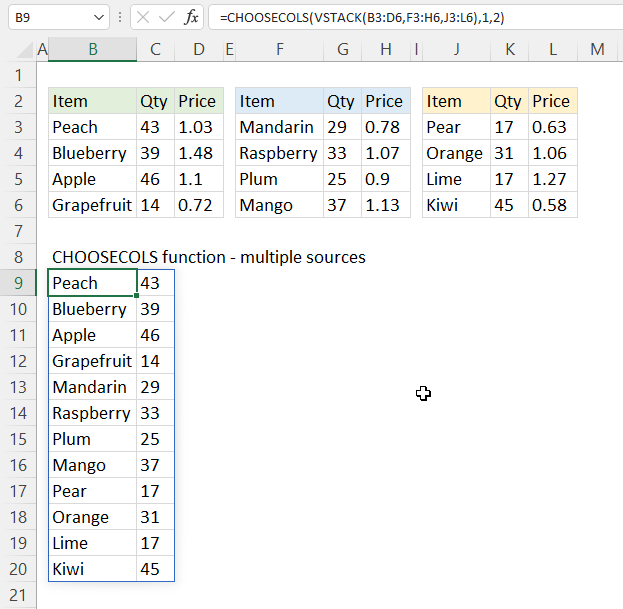The picture above shows a formula that merges three non-contiguous cell ranges and shows rows 1, 5, and 9.

Dynamic array formula in cell B9:

=CHOOSECOLS(VSTACK(B3:D6, F3:H6, J3:L6), 1, 2)

### 9.1 Explaining formula

#### Step 1 - Stack values horizontally

The VSTACK function lets you combine cell ranges or arrays, it joins data to the first blank cell at the bottom of a cell range or array (vertical stacking)

VSTACK(array1, [array2],...)

VSTACK(B3:D6, F3:H6, J3:L6)

becomes

VSTACK({"Peach", 43, 1.03;"Blueberry", 39, 1.48;"Apple", 46, 1.1;"Grapefruit", 14, 0.72}, {"Mandarin", 29, 0.78;"Raspberry", 33, 1.07;"Plum", 25, 0.9;"Mango", 37, 1.13}, {"Pear", 17, 0.63;"Orange", 31, 1.06;"Lime", 17, 1.27;"Kiwi", 45, 0.58})

and returns

{"Peach", 43, 1.03;
"Blueberry", 39, 1.48;
"Apple", 46, 1.1;
"Grapefruit", 14, 0.72;
"Mandarin", 29, 0.78;
"Raspberry", 33, 1.07;
"Plum", 25, 0.9;
"Mango", 37, 1.13;
"Pear", 17, 0.63;
"Orange", 31, 1.06;
"Lime", 17, 1.27;
"Kiwi", 45, 0.58}

#### Step 2 - Filter given rows

CHOOSECOLS(VSTACK(B3:D6, F3:H6, J3:L6),1, 2)

becomes

CHOOSECOLS({"Peach", 43, 1.03;
"Blueberry", 39, 1.48;
"Apple", 46, 1.1;
"Grapefruit", 14, 0.72;
"Mandarin", 29, 0.78;
"Raspberry", 33, 1.07;
"Plum", 25, 0.9;
"Mango", 37, 1.13;
"Pear", 17, 0.63;
"Orange", 31, 1.06;
"Lime", 17, 1.27;
"Kiwi", 45, 0.58}, 1, 5, 9)

and returns

{"Peach", 43;
"Blueberry", 39;
"Apple", 46;
"Grapefruit", 14;
"Mandarin", 29;
"Raspberry", 33;
"Plum", 25;
"Mango", 37;
"Pear", 17;
"Orange", 31;
"Lime", 17;
"Kiwi", 45}